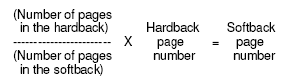# APPENDIX A — FINDING THE QUOTES IN SOFTBACK EDITIONS

To assist the reader in locating a particular quoted text, the page number and line number from the Random House hardback edition is included with each quote, represented as "page-dot-line". For example, the first line of the book is (3.1), meaning page 3, line 1, while the last line of the book is (1168.40), meaning page 1168, line 40.

From this notation, it's also possible to mathematically derive the location of the same quotes in other editions of the book. To glean an approximate page number, divide the number of pages in the hardback version, 1168, by the number of pages in any other edition, then multiply the result by the desired page number. In general, the formula appears as follows:For example, the line, "Get the hell out of my way!" appears at 1125.24 in the hardback edition. To find it in the Penguin 35th anniversary edition, which has 1074 pages, calculate 1168/1074 X 1125 = 1034. While the quote actually resides at (1035.6), the formula at least indicates the near neighborhood of the quote. In a thousand-page-plus book, this can be a true Godsend.
Be warned that this formula only gives a general answer, and in some cases the result may vary significantly from the actual location of the quote, especially for references toward the front of the book. That's because different versions have differing amounts of blank pages between the parts, and they tend to begin on pages other than Page One, such as page 3 for the hardback and page 11 for the 35th anniversary softback. As an example of this drift, the nationalization of the San Sebastian mines is reported at (72.10) in the hardback edition. Calculating 1168/1074 X 72 yields 78, but the same event in the softback edition is actually at (73.34). It's close, but not close enough.

Fortunately, a more-precise formula can be derived by taking the variations of various editions into account. First, normalize the number of pages to make the references based upon page 1. For the hardback edition (which begins on page 3), that means subtracting 2 from the number of hardback pages ( 3 - 2 = 1). Similarly, subtract 10 from the number of pages in the softback edition (which begins on page 11, so 11 - 10 = 1). To keep the page numbers consistent with each other, a similar normalization should also be performed with all page number references in the hardback edition. That means subtracting two from all page references before performing any calculations, and similarly, the final figure must then be de-normalized on the back end by adding back the ten originally subtracted.

Using this modified formula, the results for the two examples come very close to the actual location of the quote:

[ (1168-2) / (1074-10) X (1125 - 2) ] + 10 = 1034

[ (1168-2) / (1074-10) X (72 - 2) ] + 10 = 74

To gain even more accuracy, the line number can be incorporated into the calculations. Since there are 46 lines per page in the hardback edition, an approximate fractional location of the line on the page can be derived merely by multiplying the line number by 2, and including it with the page number as an actual decimal. Further, to account for the difference of white space between part 1 and part 2, subtract 1 from all results for page numbers less than 339. Using this more-precise formula, the results for the two examples come even closer:

[ (1168-2) / (1074-10) X (1125.48 - 2) ] + 10 = 1035.22

...with the .22 indicating line 11. Similarly...

[ (1168-2) / (1074-10) X (72.20 - 2) ] + 10 - 1 = 73.06

...with the .06 indicating line 3. In both cases, the result is very close to the actual location of each quote at (1035.6) and (73.34), respectively.

To sum up, the general formula is:

{ [ (HT-HN) / (ST-SN) ] X [HP-HN+(2 X HL) ] }+SN-1 = SP

where:

- HT is the total number of pages in the hardback edition

- HN is the positive difference between 1 and the first page number of the hardback edition

- ST is the total number of pages in the softback edition

- SN is the positive difference between 1 and the first page number of the softback edition

- HP is the page number of a quote in the hardback edition

- HL is the line number of a quote in the hardback edition

- SP is the page number of a quote in the softback edition

- Note that the final "- 1" is only subtracted for HP less than 339.

In general, the formula will bring you within a page of your intended destination - and that sure beats thumbing through a thousand pages! Happy hunting.

# Atlas Flubbed

Introduction
Part 1: Errors of Grammar
Part 2: Errors of Calculation
Part 3: Errors of Logic
Part 4: Errors of Philosophy
>Appendix: Finding quotes in other editions

Atlas Flubbed in PDF pamphlet format

# ATLAS  SNUBBEDAn UnsanctionedPastiche Parody